# Lagrangian of a centrifugal regulator

• dynamicskillingme
If θ increases, both the spring potential energy and the gravitational potential energy increase.(3) The distance from the pivot to the center of mass of a ball.f

## Homework Statement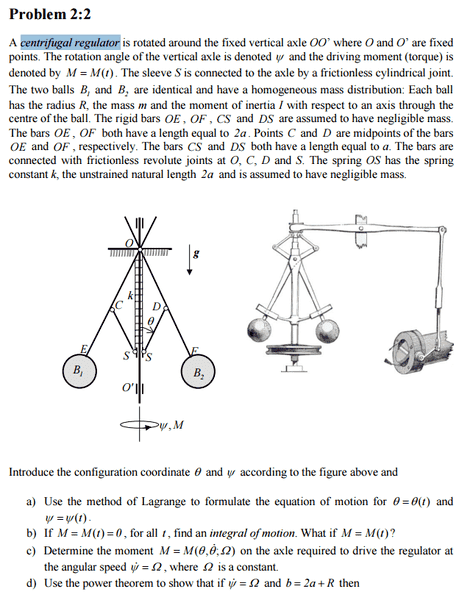L = T-V

## The Attempt at a Solution

I got a forumla for the lagrangian as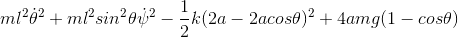I got a forumla for the lagrangian as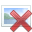check the L written above and set up the Lagrange's equations of motion...

•dynamicskillingme
check the L written above and set up the Lagrange's equations of motion...
Its more I don't know if the L is correct and wanted to check what the derivation is with someone else's because I'm pretty sure my derivation is wrong.

Its more I don't know if the L is correct and wanted to check what the derivation is with someone else's because I'm pretty sure my derivation is wrong.

the lagrangian is T- V , where T is kinetic energy and V is potential energy of the system.
The choice of generalized coordinate is important- you may consult introductory book /material on the Langrangian Method and try to set up equations for simple system like a conical pendulum and then move on to the present problem .
you can get a short introduction in the following;
http://ocw.mit.edu/courses/aeronaut...namics-spring-2003/lecture-notes/lecture7.pdf

•dynamicskillingme
Its more I don't know if the L is correct and wanted to check what the derivation is with someone else's because I'm pretty sure my derivation is wrong.

your regulator has two degrees of freedom one described by angle si and the other by angle theta.
so the kinetic energy can be written using the rotational motion about si and about theta.if you take vertical axis as z then the other motion is in x-y plane and can be described by time rate of change of theta .
the potential energy can be written using the position of two messes at any theta.

•dynamicskillingme
Its more I don't know if the L is correct and wanted to check what the derivation is with someone else's because I'm pretty sure my derivation is wrong.
You have neglected the rotational inertia of the spheres about their centers. This will add additional terms to T. Also, check the sign of the gravitational potential energy part of L.

•dynamicskillingme
I don't believe the balls are allowed to rotate about their centers. Why wouldn't the gravitational potential be positive? Also this might just be me being ill but are these the correct L eq's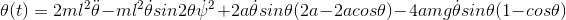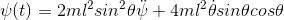I don't believe the balls are allowed to rotate about their centers.
Each ball does rotate as either ##\theta## or ##\psi## changes. Consider a ball attached to the end of a stick as shown in the figure below. As the stick rotates through an angle θ, the ball also rotates through θ.
Why wouldn't the gravitational potential be positive?
The potential energy is subtracted in the Lagrangian: L = T - V. You got the sign correct for the spring potential energy.

#### Attachments

•dynamicskillingme
Yeah I agree with that, but what other term would that introduce?
Also wouldn't the gravity term be the opposite direction to the spring?

Yeah I agree with that, but what other term would that introduce?
It's just a kinetic energy term due to rotation about the center of mass.
Also wouldn't the gravity term be the opposite direction to the spring?
If θ increases, both the spring potential energy and the gravitational potential energy increase.

•dynamicskillingme
Okay so what would the term be?

Check your text, or do a web search on "rotational kinetic energy". It involves the moment of inertia and angular speed.

Last edited:
•dynamicskillingme
In the potential energy of gravity term, the distance ##2a## is not quite the correct distance. You need to use the distance from the pivot ##O## to the center of mass of a ball.

In your Lagrangian you use the symbol ##l##. What does that stand for?

•dynamicskillingme
Could you possibly write out the correct Lagrangian as I feel like I'm stumbling around it and would love to inspect the correct one. And sorry there is a note at the start of my work I forgot and it just says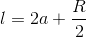Could you possibly write out the correct Lagrangian as I feel like I'm stumbling around it and would love to inspect the correct one. And sorry there is a note at the start of my work I forgot and it just saysThis is not quite the correct expression for ##l##. The ##R/2## part is incorrect. ##l## should be the distance from ##O## to the center of mass of one of the balls.

I won't write out what I get for the Lagrangian since this is your assignment. I have pointed out a few places where your expression for the Lagrangian is either incomplete or incorrect:

(1) Your first two terms represent the kinetic energy due to motion of the center of mass of the balls. In addition, you need to include terms representing the kinetic energy due to rotation of the balls about their center of mass as ##\theta## and ##\psi## change.
(2) The overall sign of the gravitational energy term needs correction.
(3) In the gravity term, you have not used the correct distance from ##O## to the center of mass of a ball.

To help with (1), consider how you would write the kinetic energy of a sphere of moment of inertia ##I## rotating with angular speed ##\omega## about an axis through its center.

Last edited:
•dynamicskillingme
Boom, found some worked solutions to give me tips along what I could be missing.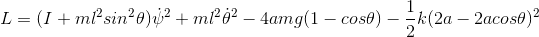That goes to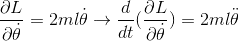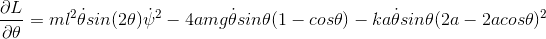and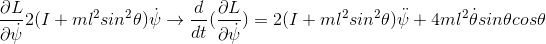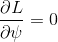(please be right, also I just saw the gravity term isn't right but its late. will change)That's getting very close to the correct ##L##. However, you need to include a rotational KE term associated with ##\dot{\theta}## as well as with ##\dot{\psi}##. And, as you say, the coefficient for the gravity terms needs to be corrected.When evaluating ##\frac{\partial{L}}{\partial \theta}##, how did you get factors of ##\dot{\theta}## to appear?

I believe your expression for ##\frac{\partial{L}}{\partial \dot\psi}## is correct. In ##\frac{d}{dt}\frac{\partial{L}}{\partial \dot\psi}##, you dropped a factor of ##\dot\psi## the last term.

•dynamicskillingme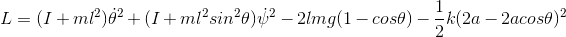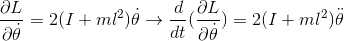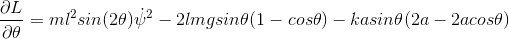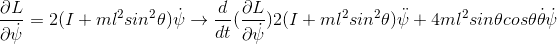MaybeThis expression for ##L## looks correct to me as long as the distance ##l## has the correct interpretation.

Everything else looks good except for your result for ##\frac{\partial L}{\partial \theta}## :.
The gravity term is not correct and I think you might have dropped a factor of 2 in the last term.

•dynamicskillingme
Praise the gods!, I changed the factor from 4 to 2 because I put l instead of 2a (which was meant to be 2a+R). Thank you so much for your help on this thread! its crazy how much you can forget between academic years!